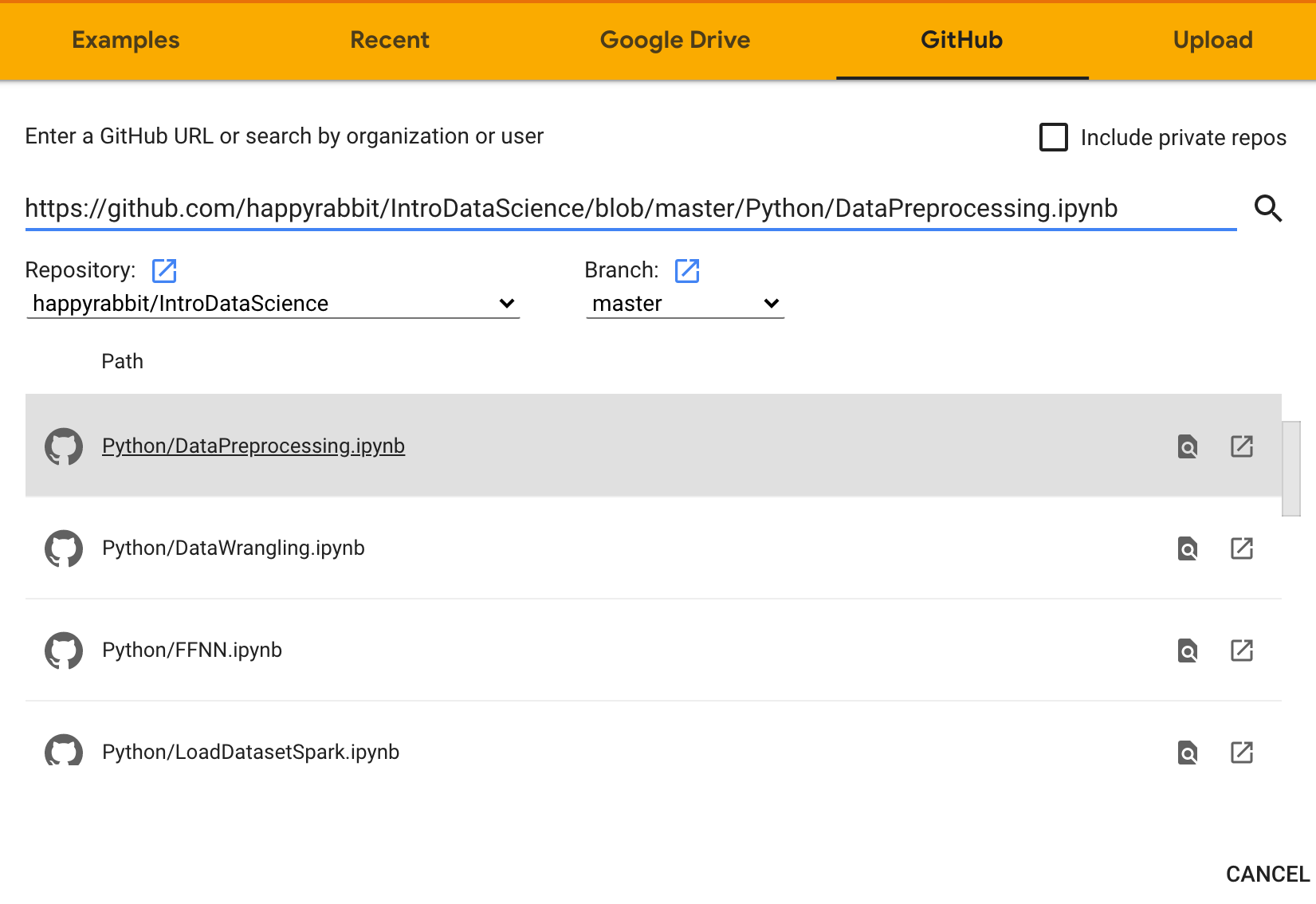## How to Use This Book

### What the Book Assumes

The first two chapters do not have any prerequisite, and the rest of the chapters do require R or Python programming experience and undergraduate level statistics. This book does NOT try to teach the readers to program in the basic sense. It assumes that readers have experience with R or Python. If you are new to the programming languages, you may find the code obscure. We provide some references in the Complementary Reading section that can help you fill the gap.

For some chapters (5, 7 - 12), readers need to know elementary linear algebra (such as matrix manipulations) and understand basic statistical concepts (such as correlation and simple linear regression). While the book is biased against complex equations, a mathematical background is good for the deep dive under the hood mechanism for advanced topics behind applications.

### How to Run R and Python Code

This book uses R in the main text and provides most of the Python codes on GitHub.

Use R code. You should be able to repeat the R code in your local R console or RStudio in all the chapters except for Chapter 4. The code in each chapter is self-sufficient, and you don’t need to run the code in previous chapters first to run the code in the current chapter. For code within a chapter, you do need to run from the beginning. At the beginning of each chapter, there is a code block for installing and loading all required packages. We also provide the .rmd notebooks that include the code to make it easier for you to repeat the code. Refer to this page http://bit.ly/3r7cV4s for a table with the links to the notebooks.

To repeat the code on big data and cloud platform part in Chapter 4, you need to use Databricks, a cloud data platform. We use Databricks because:

• It provides a user-friendly web-based notebook environment that can create a Spark cluster on the fly to run R/Python/Scala/SQL scripts.
• It has a free community edition that is convenient for teaching purpose.

Follow the instructions in section 4.3 on the process of setting up and using the spark environment.To repeat the code for big data, like running R notebook, you need to set up Spark in Databricks. Follow the instructions in section 4.3 on the process of setting up and using the spark environment. Then, run the “Create Spark Data” notebook to create Spark data frames. After that, you can run the pyspark notebook to learn how to use pyspark.